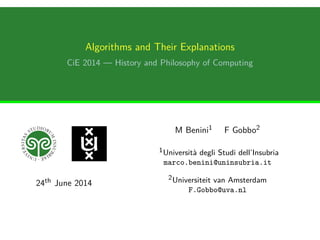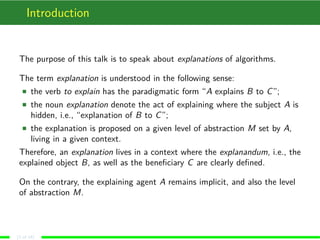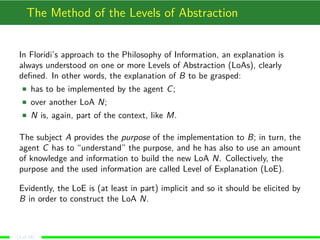Successfully reported this slideshow.

# Algorithms and Their Explanations

1

Share×
1 of 18
1 of 18

# Algorithms and Their Explanations

1

Share

By analysing the explanation of the classical heapsort algorithm via the method of levels of abstraction mainly due to Floridi, we give a concrete and precise example of how to deal with algorithmic knowledge. To do so, we introduce a concept already implicit in the method, the ‘gradient of explanations’. Analogously to the gradient of abstractions, a gradient of explanations is a sequence of discrete levels of explanation each one refining the previous, varying formalisation, and thus providing progressive evidence for hidden information. Because of this sequential and coherent uncovering of the information that explains a level of abstraction—the heapsort algorithm in our guiding example—the notion of gradient of explanations allows to precisely classify purposes in writing software according to the informal criterion of ‘depth’, and to give a precise meaning to the notion of ‘concreteness’.

By analysing the explanation of the classical heapsort algorithm via the method of levels of abstraction mainly due to Floridi, we give a concrete and precise example of how to deal with algorithmic knowledge. To do so, we introduce a concept already implicit in the method, the ‘gradient of explanations’. Analogously to the gradient of abstractions, a gradient of explanations is a sequence of discrete levels of explanation each one refining the previous, varying formalisation, and thus providing progressive evidence for hidden information. Because of this sequential and coherent uncovering of the information that explains a level of abstraction—the heapsort algorithm in our guiding example—the notion of gradient of explanations allows to precisely classify purposes in writing software according to the informal criterion of ‘depth’, and to give a precise meaning to the notion of ‘concreteness’.

## More Related Content

### Related Books

Free with a 14 day trial from Scribd

See all

### Related Audiobooks

Free with a 14 day trial from Scribd

See all

### Algorithms and Their Explanations

1. 1. Algorithms and Their Explanations CiE 2014 — History and Philosophy of Computing 24th June 2014 M Benini1 F Gobbo2 1Università degli Studi dell’Insubria marco.benini@uninsubria.it 2Universiteit van Amsterdam F.Gobbo@uva.nl
2. 2. Introduction The purpose of this talk is to speak about explanations of algorithms. The term explanation is understood in the following sense: the verb to explain has the paradigmatic form “A explains B to C”; the noun explanation denote the act of explaining where the subject A is hidden, i.e., “explanation of B to C”; the explanation is proposed on a given level of abstraction M set by A, living in a given context. Therefore, an explanation lives in a context where the explanandum, i.e., the explained object B, as well as the beneﬁciary C are clearly deﬁned. On the contrary, the explaining agent A remains implicit, and also the level of abstraction M. (2 of 18)
3. 3. The Method of the Levels of Abstraction In Floridi’s approach to the Philosophy of Information, an explanation is always understood on one or more Levels of Abstraction (LoAs), clearly deﬁned. In other words, the explanation of B to be grasped: has to be implemented by the agent C; over another LoA N; N is, again, part of the context, like M. The subject A provides the purpose of the implementation to B; in turn, the agent C has to “understand” the purpose, and he has also to use an amount of knowledge and information to build the new LoA N. Collectively, the purpose and the used information are called Level of Explanation (LoE). Evidently, the LoE is (at least in part) implicit and so it should be elicited by B in order to construct the LoA N. (3 of 18)
4. 4. Minimal information So, ﬁxed a context, the question we want to address is: What amount of information the agent needs in order to construct the required level of abstraction N over the given one M? This is best understood looking at a concrete example: the Heapsort algorithm. It has been chosen because it is simple although not trivial, and it illustrates in a nutshell all the fundamental aspects of the above question. Also, since it is classical, there is no need to explain it in the ﬁrst place: we can focus just on the analysis of explanations. (4 of 18)
5. 5. The scenario A software house has to produce a program to solve some given problem. Its employees are the programmer who has to convert a given speciﬁcation to a working code in some programming language; the software designer who has to analyse the problem and to describe an algorithmic solution which becomes the set of speciﬁcations; the algorithm designer who takes the general speciﬁcations from the software designer and convert them into precise descriptions of what the programmer must implement. Each of these agents are part of an informational organism (inforg) which is given by the agent and the computer where the program has to be implemented. In turn, each of these inforgs has an appropriate LoA the agent acts upon. (5 of 18)
6. 6. The programmer’s view The programmer receives a speciﬁcation, in the form of an algorithm. His purpose is to code this into an executable piece of code. In our example, the speciﬁcation is the Heapsort algorithm. The programmer’s LoA is given by the programming language, in a broad sense, and by the view of the machine he has through the language. Our question becomes: What “explains” the Heapsort algorithm to the programmer, enabling him to successfully achieve his purpose? (6 of 18)
7. 7. The Heapsort algorithm Heapsort(A : array) ≡ BuildMaxHeap(A) for i ←len(A) downto 2 do exchange(A,A[i]) heapsize(A)←heapsize(A)−1 MaxHeapify(A,1) BuildMaxHeap(A : array) ≡ heapsize(A)←len(A) for i ← len(A)/2 downto 1 do MaxHeapify(A,i) left(i : N) ≡ 2i right(i : N) ≡ 2i +1 MaxHeapify(A: array,i : N) ≡ m←i l ←left(i) r ←right(i) if l ≤ heapsize(A) & A[l] A[m] then m←l if r ≤ heapsize(A) & A[r] A[m] then m←r if m = i then exchange(A[i],A[m]) MaxHeapify(A,m) exchange(a: element,b: element) ≡ x ←a; a←b; b ←x (7 of 18)
8. 8. The programmer’s explanation The programmer needs to know how to read the speciﬁcation and to correctly convert it into an executable piece of code in the programming language. In other words, he has to build a new LoA, which extends the programming language with a new function, Heapsort. Thus, the minimal explanation the programmer needs of the above pseudo-code is the one which allows him to fulﬁl his task. This explanation reduces to know how to correctly interpret the pseudo-code into the programming language construction. E.g., he needs to know that ← stands for assignment. This amount of explanation suﬃces to show that the implementation exactly matches the pseudo-code, and so, it is correct with respect to the speciﬁcation the programmer is given. (8 of 18)
9. 9. The software designer’s view The purpose of the software designer is to construct a set of speciﬁcations that collectively allows to write a program that solves the problem. The software designer, in our example, has reduced a part of the solution to the need of sorting an array. So, he has to understand what are the features this sorting algorithm must have. For example, he has to decide that stability does not matter, while eﬃciency is important. For a software designer, an algorithm is not a procedure, but a collection of properties. He has to decide what properties are relevant to solve the problem, and to pass them to the algorithm designer, who converts them into pieces of pseudo-code, and then, to the programmer, who convert the pseudo-code into an executable program. (9 of 18)
10. 10. The software designer’s explanation After identifying sorting as a (sub-)problem to be solved, the software designer has to decide what the sorting procedure should actually do. For him, the “right” sorting procedure must exhibit some features, while it may not have others because irrelevant to the solution he designs. Thus, the explanation of HeapSort is, for him, a collection of properties: it sorts an array; it rearranges the input, instead of generating a sorted copy; it is not stable; it is eﬃcient among comparison-based sorting algorithms. This explanation allows the software designer to show that the solution he provides is correct, i.e., it solves the original problem within the given constraints. Note how the software designer does not need to know how Heapsort operates! (10 of 18)
11. 11. The algorithm designer’s view As the software designer is interested in “what” an algorithm does, the algorithm designer focuses on “how” an algorithm achieves its result. In our example, the algorithm designer may think that sorting data in a tree is more eﬃcient than sorting data in a random-access sequence. Of course, this is true when the tree satisﬁes some conditions, like that each node’s key is less than the parent’s. Understanding that an array can be interpreted as a tree, allows to write an algorithm that does not use an additional data structure. In this way, eventually, the algorithm designer will be able to prove that the designed pseudo-code, which is the previously shown Heapsort procedure, is correct, that is, it sorts an array. Also, destructive manipulation of the input and lack of stability immediately follow from the correctness proof. Showing that Heapsort is eﬃcient requires to reduce the execution time, measured as number of computational steps, to a set of mutually recursive equations. And the solution of these equations clearly shows that Heapsort operates in O(nlogn) with n the length of the array. (11 of 18)
12. 12. The algorithm designer’s explanation So, the algorithm designer is able to show that his pseudo-code, the LoA he constructed, satisﬁes the speciﬁcation he received. To provide this evidence, he has to show that the pseudo-code sorts an array and do it within the constraints put by the software designer, which amounts to explain how the computational process he designed eventually produces the output; to calculate the complexity of the algorithm, which amounts to deduce from the algorithm and to solve a set of recurrence equations. The knowledge and information to obtain these two goals are essential to justify his work, so this is the minimal explanation which suits the algorithm designer’s purpose. (12 of 18)
13. 13. The Minimal Levels of Abstraction When the purpose has been accomplished, the resulting machine shows (at least) two levels of abstraction: 1. the LoA which has been used to implement Heapsort over it; 2. the LoA which has Heapsort as a primitive. Each of these LoAs can be described within Floridi’s framework, thus being in strict interaction with a corresponding level of observation, which enables us to measure information. Although very simple, these two LoAs provide a gradient of abstractions: the description of the system used by the software house of our example is, really, the gradient, i.e., the sum of the two LoAs together with their mutual interactions. (13 of 18)
14. 14. The Levels of Explanation of an Algorithm The Heapsort procedure as implemented can be explained in, at least, four diﬀerent ways: 1. to the machine, it is explained by the operational semantics, encoded in the computer machinery, which allows to execute the code. 2. to the programmer, it is explained as the adherence to the pseudo-code; 3. to the system designer, it is explained as a set of properties that allows the procedure to take its role inside the program; 4. to the algorithm designer, it is explained as an algorithm which solves a useful problem, and which enjoys a number of “good” properties; Each Level of Explanation (LoE) allows to fulﬁl a speciﬁc purpose, the one of the explaining agent. In the last three cases this has been already illustrated, while in the ﬁrst, the purpose is simply to execute the procedure on a given input. (14 of 18)
15. 15. A gradient of explanation In Floridi’s terms, each complex, structured information, is understood on one of more Levels of Abstractions (LoAs), where each one presumes a Level of Explanation (LoE) that is used to construct what we informally called explanation. And, the four explanations are strictly linked one to the others, together providing an explanation for (part of) the purpose of the software house: to provide a program that solves a speciﬁc problem. (15 of 18)
16. 16. Minimal explanations and measures A gradient of explanations allows for a very simple way to measure distance between explanations: we say that the machine “understands” the code less than the software designer because the explanation of the machine is at level 1 in the gradient, while the software designer’s is at level 3. It is important to remark that this measure is relative to a gradient and a LoA: it does not make sense to use it outside this context. Nevertheless, this measure can be made more “objective” when we consider minimal explanations — as we tried to do — because any other gradient of explanations on the same LoA and inforgs would become an extension of the minimal one. (16 of 18)
17. 17. Conclusion The concept of the Gradient of Explanations (GoE) presented here was naturally derived within the Method of the LoAs. It is important to note, that the GoE has a diﬀerent epistemological status compared to the Gradient of Abstractions (GoA). The consequences of the introduction of the GoE are not yet fully explored, and its formalisation is still preliminary. However, the Heapsort example will act as a concrete guideline so to apply the GoE in other cases. (17 of 18)
18. 18. Thank you for your attention! (18 of 18)